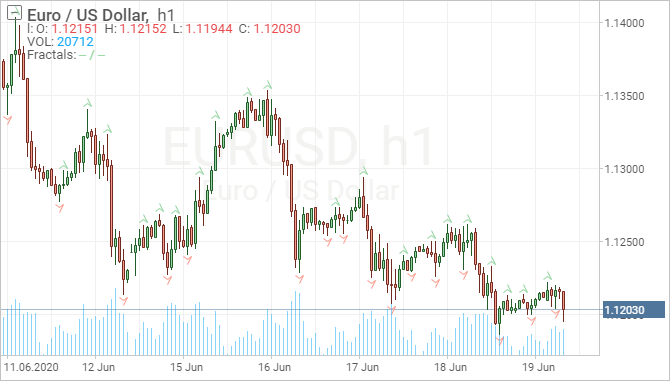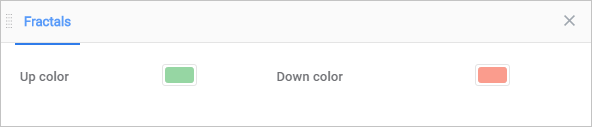Overview

The Fractal indicator is based on a simple price pattern that is frequently seen in financial markets. Outside of trading, a fractal is a recurring geometric pattern that is repeated on all time frames. From this concept, the fractal indicator was devised. The indicator isolates potential turning points on a price chart. It then draws arrows to indicate the existence of a pattern. The bullish fractal pattern signals the price could move higher. A bearish fractal signals the price could move lower. Bullish fractals are marked by a down arrow, and bearish fractals are marked by an up arrow.KEY TAKEAWAYS

• A bullish fractal occurs when there is a low point with two higher low bars/candles on each side of it.
• A bearish fractal occurs when there is a high point with two lower high bars/candles on each side of it.
• An up arrow marks the location of a bearish fractal, while a down arrow marks the location of a bullish fractal.
• Arrows are drawn above or below the middle bar (high or low point), even though the pattern is five bars. There is no way a trader could enter a trade at the arrow because the arrow only occurs if the next two bars create the pattern.
• If someone were to trade fractal signals, the entry would be the open price of the third bar after the arrow.

Description

The Fractal indicator will generate signals frequently. The existence of a fractal isn't necessarily important since the pattern is so common.

The fractal is indicating the possibility of a trend change. This is because fractals are essentially showing a "U-shape" in price. A bearish fractal has the price moving upward and then downward, forming an upsidedown U. A bullish fractal occurs when the price is moving down but then starts to move up, forming a U.

Because fractals occur so frequently, and many of the signals aren't reliable entry points, fractals are typically filtered using some other form of technical analysis. Bill Williams also invented the alligator indicator which isolates trends. By combining fractals with trend analysis, a trader may decide to only trade bullish fractals signals while the price trend is up. If the trend is down they may take only short trades on bearish fractal signals, for example.

Fractals could also be used with other indicators, such as pivot points or Fibonacci retracement levels. A fractal is only acted on if it aligns with one of these other indicators and potentially the longer-term price direction. For example, assume a stock is trending higher. The price is pulling back and reaches a 50% Fibonacci retracement level. Since the trend is up, and the price is near a Fibonacci retracement level, the trader will take a trade if a bullish fractal forms.